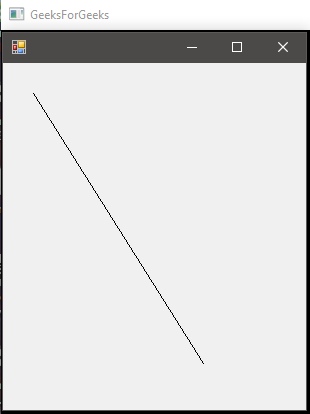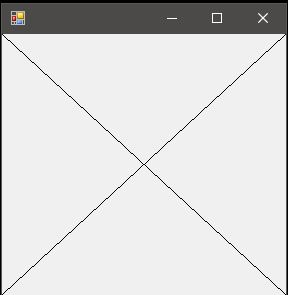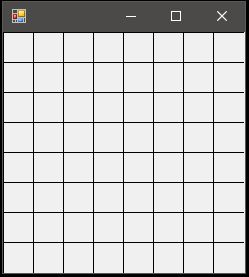# C# | Graphics.DrawLine() Method | Set – 1

Graphics.DrawLine() Method is used to draw a line connecting the two points specified by the coordinate pairs. There are 4 methods in the overload list of this method as follows:

• DrawLine(Pen, PointF, PointF) Method
• DrawLine(Pen, Int32, Int32, Int32, Int32) Method
• DrawLine(Pen, Single, Single, Single, Single) Method
• DrawLine(Pen, Point, Point) Method

Here, we will discuss the first two methods.

#### DrawLine(Pen, PointF, PointF) Method

This method is used to draw line form a specified set of points to a specified set of points. It needs a PointF variable which is set of (x, y) points.

Syntax:

public void DrawLine (System.Drawing.Pen pen, System.Drawing.PointF pt1, System.Drawing.PointF pt2);

Parameters:

• pen: Pen determines the color, width, and style of the line.
• pt1: Defines the (x, y) coordinates as a PointF variable of the initial point.
• pt2: Defines the (x, y) coordinates as a PointF variable of the final point.

Exception: This method will give ArgumentNullexception if the pen is null.

Example:

 `// C# program to demonstrate the  ` `// DrawLine(Pen, PointF, PointF) Method ` `using` `System; ` `using` `System.Drawing; ` `using` `System.Drawing.Printing; ` `using` `System.Windows.Forms; ` ` `  `namespace` `GFG { ` ` `  `class` `PrintableForm : Form { ` ` `  `    ``public` `static` `void` `Main() ` `    ``{ ` `        ``Application.Run(``new` `PrintableForm()); ` `    ``} ` ` `  `    ``public` `PrintableForm() ` `    ``{ ` `        ``ResizeRedraw = ``true``; ` `    ``} ` `    ``protected` `override` `void` `OnPaint(PaintEventArgs pea) ` `    ``{ ` `        ``// Defines pen ` `        ``Pen pen = ``new` `Pen(ForeColor); ` ` `  `        ``// Defines the both points to connect ` `        ``// pt1 is (30.0, 30.0) which represents (x1, y1) ` `        ``PointF pt1 = ``new` `PointF(30.0F, 30.0F); ` ` `  `        ``// pt2 is (200.0, 300.0) which represents (x2, y2) ` `        ``PointF pt2 = ``new` `PointF(200.0F, 300.0F); ` ` `  `        ``// Draws the line ` `        ``pea.Graphics.DrawLine(pen, pt1, pt2); ` `    ``} ` `} ` `} `

Output:#### DrawLine(Pen, Int32, Int32, Int32, Int32) Method

This method is used to draw line form a specified set of coordinates given in form x1, y1, x2, y2 all discrete.

Syntax:

public void DrawLine (System.Drawing.Pen pen, int x1, int y1, int x2, int y2);

Parameters:

• pen: Pen determines the color, width, and style of the line.
• x1: The abscissa of first point.
• y1: The ordinate of first point.
• x2: The abscissa of second point.
• y2: The ordinate of second point.

Exception: This method will give ArgumentNullexception if the pen is null.

Example 1:

 `// C# program to draw a cross using the ` `// DrawLine(Pen, Int32, Int32, Int32, ` `// Int32) Method ` `using` `System; ` `using` `System.Drawing; ` `using` `System.Drawing.Printing; ` `using` `System.Windows.Forms; ` ` `  `namespace` `GFG { ` ` `  `class` `PrintableForm : Form { ` ` `  `    ``public` `static` `void` `Main() ` `    ``{ ` `        ``Application.Run(``new` `PrintableForm()); ` `    ``} ` ` `  `    ``public` `PrintableForm() ` `    ``{ ` `        ``ResizeRedraw = ``true``; ` `    ``} ` ` `  `    ``protected` `override` `void` `OnPaint(PaintEventArgs pea) ` `    ``{ ` `        ``// Defines the pen ` `        ``Pen pen = ``new` `Pen(ForeColor); ` ` `  `        ``// To draw a cross we need to make 2  ` `        ``// diagonals from top-left to the  ` `        ``// bottom-right and top-right to the  ` `        ``// bottom-left to calculate these  ` `        ``// coordinates we would take help of  ` `        ``// our screen size top-left = (0, 0) ` `        ``// bottom-right = (ClientSize.Width - 1, ` `        ``// ClientSize.Height - 1) ` `        ``// top-right = (ClientSize.Width - 1, 0) ` `        ``// bottom-left = (0, ClientSize.Height - 1) ` `        ``pea.Graphics.DrawLine(pen, 0, 0, ClientSize.Width - 1, ` `                                       ``ClientSize.Height - 1); ` ` `  `        ``pea.Graphics.DrawLine(pen, ClientSize.Width - 1, 0, 0, ` `                                       ``ClientSize.Height - 1); ` `    ``} ` `} ` `} `

Output:Example 2:

 `// C# program to Create a 8x8 square board ` `// using // DrawLine(Pen, Int32, Int32, Int32, ` `// Int32) Method ` `using` `System; ` `using` `System.Drawing; ` `using` `System.Drawing.Printing; ` `using` `System.Windows.Forms; ` ` `  `namespace` `GFG { ` ` `  `class` `PrintableForm : Form { ` ` `  `    ``public` `static` `void` `Main() ` `    ``{ ` `        ``Application.Run(``new` `PrintableForm()); ` `    ``} ` ` `  `    ``public` `PrintableForm() ` `    ``{ ` `        ``ResizeRedraw = ``true``; ` `    ``} ` ` `  `    ``protected` `override` `void` `OnPaint(PaintEventArgs pea) ` `    ``{ ` `        ``// Define Pen ` `        ``Pen pen = ``new` `Pen(ForeColor); ` ` `  `        ``// loop for all 7 horizontal lines to draw ` `        ``for` `(``int` `i = 0; i < 7; i++)  ` `        ``{ ` `            ``// Taking Block size to be 30x30 ` `            ``// So width and height will be 30*8=240 ` `            ``pea.Graphics.DrawLine(pen, i * 30, 0, i * 30, 240); ` `        ``} ` ` `  `        ``// loop for all 7 horizontal lines to draw ` `        ``for` `(``int` `i = 0; i < 7; i++)  ` `        ``{ ` `            ``pea.Graphics.DrawLine(pen, 0, i * 30, ` `                                    ``240, i * 30); ` `        ``} ` `    ``} ` `} ` `} `

Output:My Personal Notes arrow_drop_upCheck out this Author's contributed articles.

If you like GeeksforGeeks and would like to contribute, you can also write an article using contribute.geeksforgeeks.org or mail your article to contribute@geeksforgeeks.org. See your article appearing on the GeeksforGeeks main page and help other Geeks.

Please Improve this article if you find anything incorrect by clicking on the "Improve Article" button below.

Article Tags :

Be the First to upvote.

Please write to us at contribute@geeksforgeeks.org to report any issue with the above content.